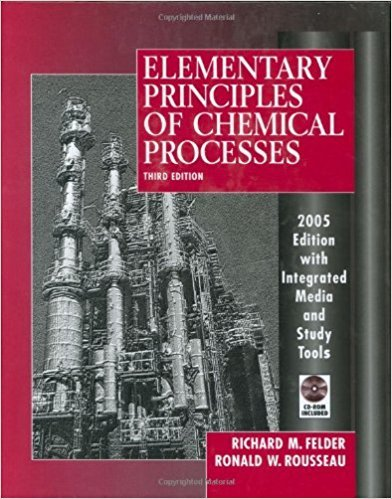×
Get Full Access to Elementary Principles Of Chemical Processes - 3 Edition - Chapter 7 - Problem 7.51
Get Full Access to Elementary Principles Of Chemical Processes - 3 Edition - Chapter 7 - Problem 7.51

×

# A liquid mixture of benzene and toluene is to be separatedISBN: 9780471687573 143

## Solution for problem 7.51 Chapter 7

Elementary Principles of Chemical Processes | 3rd Edition

• Textbook Solutions
• 2901 Step-by-step solutions solved by professors and subject experts
• Get 24/7 help from StudySoup virtual teaching assistantsElementary Principles of Chemical Processes | 3rd Edition

4 5 1 240 Reviews
12
2
Problem 7.51

A liquid mixture of benzene and toluene is to be separated in a continuous single-stage equilibrium flash tank. Vapor product Liquid feed 1 mol (basis) T(OC) P(mm Hg) nv(mol) ys(mol C6H61mol) The pressure in the unit may be adjusted to any desired value. and the heat input may similarly be adjusted to vary the temperature at which the separation is conducted. The vapor and liquid product streams both emerge at the temperature T (0C) and pressure P(mm Hg) maintained in the vessel. Assume that the vapor pressures of benzene and toluene are given by the Antoine equation. Table 6.1-1; that Raoult's law-Equation 6.4-1-applies; and that the enthalpies of benzene and toluene liquid and vapor are linear functions of temperature. Specific enthalpies at two temperatures are given here for each substance in each phase. C6H6 (l) C6H6 (v) C7H8(l) C7H8(v) (T = Oe, fJ = 0 kllmol) (T = 80C, fJ = 41.61 kllmol) (T = ooe, fJ = 0 kllmol) (T = 89C, fJ = 49.18 kllmol) (T = 80e, fJ = 10.85 kllmol) (T = 120e, fJ = 45.79 kllmol) (T = 111e,fJ = 18.58kJ/mol) (T = 111e,fJ = 52.05kllmol) (a) Suppose the feed is equimolar in benzene and toluene (;:8 = 0.500). Take a basis of 1 mol offeed and do the degree-of-freedom analysis on the unit to show that if T and P are specified. you can calculate the molar compositions of each phase (XB and YB). the moles of the liquid and vapor products (nL and ny). and the required heat input (Q). Don't do any numerical calculations in this part. (b) Do the calculations of part (a) for T = 90C and P = 652 mm Hg. (Suggestion: First derive an equation for XB that can be solved by trial and error from known values of T and P.) (c) For Zs = 0.5 and T = 90C, there is a range of feasible operating pressures for the evaporator. Pmin < P < Pmax If the evaporator pressure P fell outside this range, no separation of benzene and toluene would be achieved. Why not? What would emerge from the unit if P < Pmin : What would emerge if P > Pmax? [Hint: Look at your solution to part (b) and think about how it would change if you lowered P.] *(d) Set up a spreadsheet to perform the calculation of part (b) and then use it to determine Pmat and Pmin The spreadsheet should appear as follows (some solutions are shown): 1 0))4.-' I 8 1 7.51-Flash vaporization of benzene and toluene I I I I I I I I , I I I i i , : zB T , P pB* I pT* xB yB nL nV i Q I : : I I i I I I I i j I I 652 I 102 [ I I I I I 5 00 I -- '"' I , 44 I I , i i I i 0.500 90.0 I 714 ! ! ! -6.093 I I 90.0 I I I, I 0.500 I I I I i , *Computer problem. 354 Chapter 7 Energy and Energy Balances Additional columns may be used to store other calculated variables (e.g., specific enthalpies). Briefly explain why Qis positive when P = 652 mm Hg and negative when P = 714 mm Hg. (e) In successive rows, repeat the calculation for the same ZB and T at several pressures between Pmin and Pmax. Generate a plot (using the spreadsheet program itself, if possible) of nv versus P. At approximately what pressure is half of the feed stream vaporized?

Step-by-Step Solution:
Step 1 of 3

Ch. 14: Solutions Highlight: key formulas Highlight: key terms Highlight: important things to remember • Solution: homogeneous mixture of two or more substances (solvent & solute) o Solvent: the majority component that the solute dissolves in o Solute: the minority component that dissolves in the solvent o Solvents and solutes interact via intermolecular forces • Types of Solutions o Gaseous: Gas + Gas o Liquid: Gas + Liquid; Liquid + Liquid; Solid + Liquid o Solid: Solid + Solid • Aqueous Solution: type of solution in which water is the solvent and another substance is the solute • Solubility: measures the amount of substance that will dissolve in given amount of a solvent

Step 2 of 3

Step 3 of 3